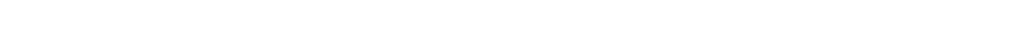# how to Get the size of a list in python

## List in Python

There are different data types in python that are capable of storing sequences of items. One of them is a list. It can be defined as an ordered collection of items that can be of different data types. In this article, we are going to learn different methods to find the size and length of a list using Python.

The length and size are two different terms. The length of a list is determined by the count of data elements inside that list. On the other hand, the size of a list is basically the number of bytes occupied in memory by the list object.

## list size

One of the most essential requirements in computer programs is memory management. When writing large codes, it is necessary to make sure there is much available memory space so that new processes can be added to it. To handle memory efficiently, we need to know how much memory is required by the objects and data structures used in the code. For this, we need to determine the size of the list.

Python has a sys module that provides the getsizeof() function. The function accepts an object and calls __sizeof()__ method which returns the number of bytes currently being used by the data structure along with the garbage collector overhead if the object is managed by the garbage collector. That is why the size of the list obtained using getsizeof() function is greater than the size acquired through __sizeof()__ method. The garbage collector is used to keep track of all the objects in the memory.

### Example 1:

```# Using Sys Module to getsizeof()

import sys

# Create and initialize list

list1 = [1, 2, 3, 4, 5]

list2 = [1, "Programming", "Entechin", 5.3]

# Print the sizes of lists

print ("Size of list 1 in bytes: ", sys.getsizeof(list1))

print ("Size of list 2 in bytes: ", sys.getsizeof(list2))

.
```

In this example, we have first created two lists as shown in the code. List1 contains 5 integers and list2 is composed of mixed datatypes. In order to determine the size of the list, we have passed the list object to the getsizeof() function which on execution return the sizes of list1 and list2 in bytes along with garbage collector overhead. List1 and list2 are occupying 112 bytes and 104 bytes in the memory.

You can also use of __sizeof_() method directly to find the size of the object. The size returned by this method is only of the object that is why it is smaller than the size determined by the getsizeof() because the latter takes overhead into account.

### Example 2:

Let’s take the same example 1 but this time determine the size of the list using __sizeof__() method.

```# Using  __sizeof__() method.

import sys

# Create and initialize list

list1 = [1, 2, 3, 4, 5]

list2 = [1, "Programming", "Entechin", 5.3]

# Print the sizes of lists

print ("Size of list 1 in bytes: ", list1.__sizeof__())

print ("Size of list 2 in bytes: ", list2.__sizeof__())

.
```The output window shows the original size of both list objects in bytes.

## Length of List

In most applications, you are required to perform the same operation on all the elements of a list or to find a certain item. So for all such applications, we need to iterate or traverse over the list. For this, you need the total length of the list.

### len() function

The python has a built-in function len() to measure the length of a list. It takes the list as an argument and returns the total number of elements in the list. It is considered to be one of the best methods to find the length of a list as it returns the length in O(1) time.

```# Using len() Function

list = ['apple',  'mango', 'orange', 'guava']

length = len(list)

print ("Length of a list: ",length)

.
```

### For Loop

For loop can also be used to calculate list length. It goes throughout the list and counts every single element in the list. After initializing a list, declare a counter variable and initialize it to zero. using for loop, traverse through the list one by one on each iteration and increment the counter variable by 1. After the last iteration, the counter will hold the length of the list.

```# Using For Loop

# Create and Initialize a list1

list1 = [ "John", "Harry", "Peter"]

# Declare a variable to store the length of list

count = 0;

for i in list1:

count = count + 1

print("Length of list is: ", count)

.
```

Output:

As the length is analogous to the count of list elements that’s why the value in the count variable represents the length of the list. The for loop can also be used along with other functions to iterate over the list which is discussed in the previous article.

Python is the most popular programming language. Size and length are often misunderstood by each other but these are two different terms. In this tutorial, we have learned the different ways to obtain the size and length of lists. If you want to learn about Python, please follow our website. Let us know if you have queries regarding this topic or if you want to use cover a certain topic. It would be highly appreciated.

For any questions related to the Size of the list in Python, feel free to Contact Us.

Automated page speed optimizations for fast site performance